# Box 2

In his 1905 paper on Special Relativity, and during later years, Albert Einstein worked out some of the main physical consequences of his theory, like length contraction, time dilation and the much celebrated formula E=mc2. After more than a hundred years from their formulation, these effects have all been experimentally verified to a high degree of precision, over and over again. As the theory was developed assuming constancy of the one-way velocity of light (see main text and Box 1), and given that this is equivalent to setting ε=1/2 in Reichenbach’s synchronization formalism (see Box 1), it would appear that Special Relativity success is a confirmation that Einstein’s convention is in fact a true property of nature and that it is perfectly feasible to consider the one-way velocity of light as a true physical quantity. Work by a renowned group of philosophers of science, known as the conventionalists, has shown this to be a misconception. In a paper published in 1970 John Winnie, from the University of Hawaii, has been able to re-formulate Special Relativity without the one-way velocity of light assumption, maintaining throughout the theory the ε synchronization parameter as undetermined, i.e. explicitly avoiding to setting it to ½. The author has calculated length contraction and time dilation with his formulation and has shown them to lead to conclusions experimentally identical to what Einstein derived in 1905. Here we will shortly described the time dilation case, because it is the basis for one of the most dramatic experimental verification of Relativity, particles decay during accelerators experiments.

Certain particles of the sub-atomic world have been observed to decay after a given time since their creation. This is true for the neutron, for muons and many other particles. In giant accelerators like those at SLAC in the States or CERN at Geneva, this decay is observed on a daily basis. Normally the decay is measured as a distance, rather than as a time interval. Consider a particle whose decay time is Δt. If the particle travels with speed v, it should decay after a length dL=vΔt. According to Special Relativity, the observed decay time, Δt’, will be different for somebody watching the particle from a measuring station. More specifically, the following relation holds: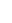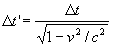(2.1)

Because the quantity inside square root is always smaller than 1, Δt’ is always greater than Δt. This is what is known as time dilation in Special Relativity; a physical process takes longer to develop if observed by a moving reference system. Thus, if Special Relativity were not true, the particle would decay after a length dL=vΔt. But, given that the observer is moving relatively to the particle, the decay distance is given by the following formula: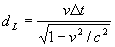(2.2)

Useless to say, the decay distance measured in all experiments performed well agrees with formula (2.2). Let us now turn to the prediction using a value different from ½ for the synchronization parameter. It can be shown that time dilation, in this case, is not given by formula (2.1) but, rather, by the following one: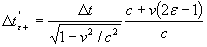(2.3)

If ½ is replaced for ε in formula (2.3), Special Relativity time dilation, equation (2.1), is found, as it should be. The fact that formula (2.3) is so different from formula (2.1) leads us to think that alternatives clocks synchronizations are definitely ruled out by experimental results, as the decay distance (2.2) cannot be derived from formula (2.3). But, if we use a different synchronization, we should expect that the particle velocity, which is a one-way velocity, is not going to be v, but rather vε+, as derived in (1.4) (Box 1). Thus, the decay distance is not vΔtε+’, but, rather, vε+Δtε+’. Using formulas (1.4) and (2.3) we have, therefore,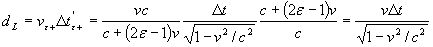This result is identical with result (2.2). The experimental verification of formula (2.2) for particles decay does not rule out different clocks synchronizations or, alternatively, it is not a confirmation that one-way velocities can be unambiguously measured.

Further readings

The paper by John Winnie mentioned above is:

John A. Winnie, “Special Relativity without one-way velocity assumptions”, Philosophy of Science 37 (1970): 81-99, 223-238

This is not an easy reading for students and it is rather suggested that teachers read it  first, in order to elicit its best approachable parts for their pupils.